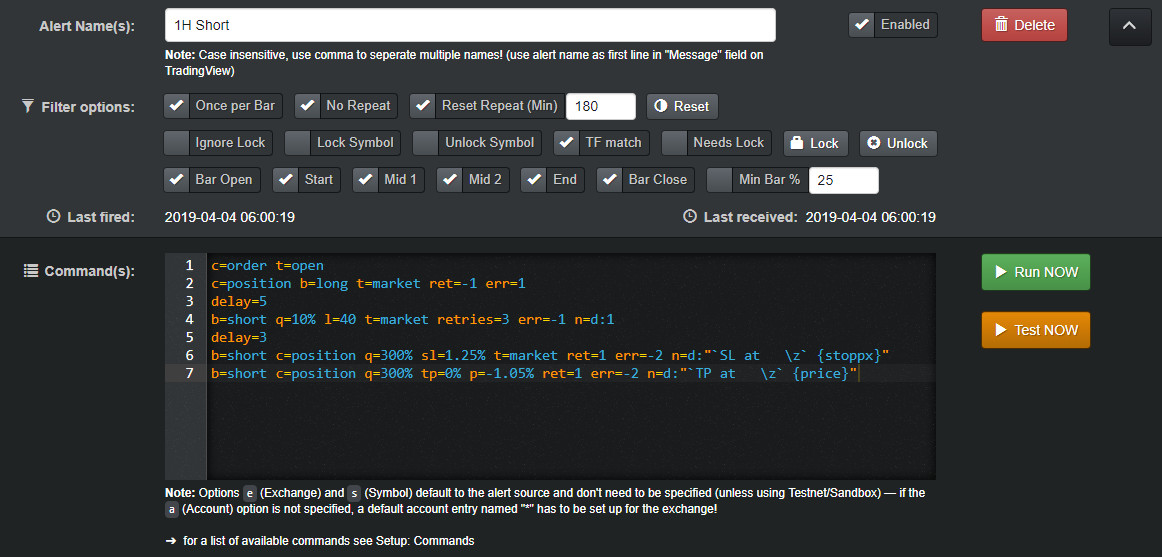#### Long Signal

``````### Bybit Long ###

# Optionally for multiple accounts uncomment the following line and fill in your accounts
#ap=acc1,acc2,acc3

# cancel all orders
c=order
# close open shorts at market, if no position skip delay
c=position b=short t=market ret=-1 err=1
delay=5

# open long at market with some % of the available balance
b=long q=YOUR_BUY_IN% l=YOUR_LEVERAGE t=market err=-1 retries=3
delay=3

# place a market stoploss order (reduce only & expect to return an order)
b=long c=position q=100% sl=-YOUR_STOP_LOSS% t=market r=1 ret=1 err=-2 retries=10 id=SL

# place a limit take profit order (reduce only & expect to return an order)
b=long c=position q=100% p=YOUR_TAKE_PROFIT% r=1 ret=1 err=-2 retries=10 id=TP
``````

`q= l= sl= p= has to be adjusted to your strategy / personal needs!`#### Syntax Explanation

Command Description
c=order t=open close open orders
c=position b=short t=market close open shorts @ market
c=position b=short t=market safety
delay=3 3 seconds delay.
b=long l=10 q=50% t=market open market long 50% @ 10x
delay=5 5 seconds delay.
b=long c=position q=300% sl=-2.5% t=market r=1 ret=1 err=-2 fixed stop loss @ 2.5% below current price. expect to place an order, otherwise close all & abort
b=long c=position q=300% tp=0% p=1.3% r=1 ret=1 err=-2 fixed take profit @ 1.3% above current price. expect to place an order, otherwise close all & abort

#### Short Signal

``````### Bybit Short ###

# Optionally for multiple accounts uncomment the following line and fill in your accounts
#ap=acc1,acc2,acc3

# cancel all orders
c=order
# close open longs at market, if no position skip delay
c=position b=long t=market ret=-1 err=1
delay=5

# open short at market with some % of the available balance
b=short q=YOUR_BUY_IN% l=YOUR_LEVERAGE t=market err=-1 retries=3
delay=3

# place a market stoploss order (reduce only & expect to return an order)
b=short c=position q=100% sl=YOUR_STOP_LOSS% t=market r=1 ret=1 err=-2 retries=10 id=SL

# place a limit take profit order (reduce only & expect to return an order)
b=short c=position q=100% p=-YOUR_TAKE_PROFIT% r=1 ret=1 err=-2 retries=10 id=TP
``````

`q= l= sl= p= has to be adjusted to your strategy / personal needs!`#### Syntax Explanation

Command Description
c=order t=open close open orders
c=position b=long t=market close open longs @ market
c=position b=long t=market safety
delay=3 3 seconds delay.
b=short l=10 q=50% t=market open market short 50% @ 10
delay=5 5 seconds delay.
b=short c=position q=300% sl=2.5% t=market r=1 ret=1 err=-2 fixed stop loss @ 2.5% above current price. expect to place an order, otherwise close all & abort
b=short c=position q=300% tp=0% p=-1.3% r=1 ret=1 err=-2 fixed stop loss @ 1.3% above current price. expect to place an order, otherwise close all & abort

#### Attention

Always double check that you are using the right syntax/alert!